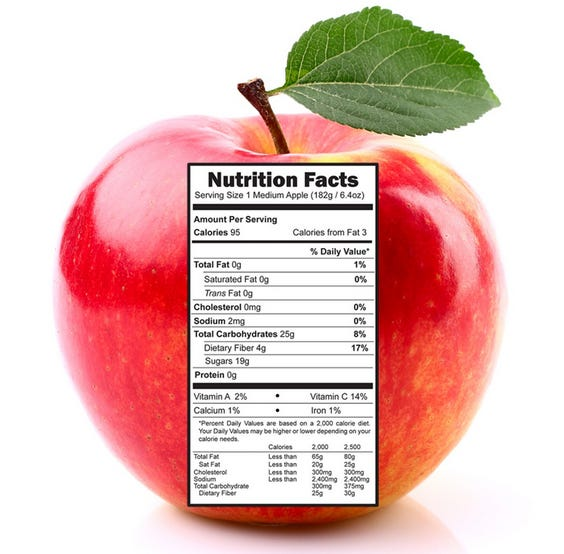# [Week 2— SeeFood]

Source: Deep Learning on Medium

Theme: Food Calorie Estimation

Team Members: Okan ALAN Gökberk Şahin Emre Yazıcısource: https://www.nutritionfacts.us/nutrition-facts-apple/

We spent this week by diving deeper into the related works we mentioned last week so we can have a better understanding of how we’re going to predict the calories of a food just from its picture.

We as humans can measure the calories of a say an apple, quite accurately. How? Well, we just need a precision balance and a smartphone to look up how many calories in an apple of 100 grams. Since we don’t have precision balances in our pockets we just eyeball the weight of the apple and multiply it by the calories/100grams. So we asked the question “how do humans eyeball?”. After reading a couple of articles online, it turns out that we tend to estimate the weight of an object by its volume and the researchers that wrote the paper mentioned in the previous post did the exact same thing. They mimicked the algorithm of how humans estimate calories. Just like humans, we will estimate the volume, then we will use this volume to estimate the mass and finally we multiply the mass by calories per grams.

Image Segmentation

The researches that wrote the paper chose Faster R-CNN instead of using semantic segmentation method such as Fully Convolutional Networks (FCN). Here, after the images are inputted as RGB channels, the researchers can get a series of bounding boxes. This process uses an image processing approach to segment each bounding box. The bounding boxes around the object that the algorithm needs can be provided by Faster R-CNN. After segmentation, we can get a series of food images stored in a matrix, but with the values of the background pixels being replaced by zeros. This will leave only the foreground pixels.

Volume Estimation

To estimate the volume, we will calculate the scale factors based on calibration objects. The researches used a 1 CNY coin to show the specific process of calculating the volume. The diameter of the coin is 2.5 cm, and the side view’s scale factor was calculated with Equation 1.

In this equation, Ws is the width of the bounding box, Hs is the height of the bounding box. Similarly, the top view’s scale can be calculated with Equation 2.

After dividing the foods into three categories based on shape: ellipsoid, column, irregular. Different volume estimation formula will be selected for different types of food, according to Equation 3. HS is the height of side view PS and LkS is the number of foreground pixels in row k (k ∈ 1,2,…,HS).

LMAX = max(Lk ,…,Lk ), it records the maximum number of foreground pixels in PS. ß is a compensation factor (default value = 1.0). After that, for each food type, there will be a unique value.

Calorie Estimation

After estimating the volume, the next step is to estimate each food’s mass. It can be calculated in Equation 4, Where v (cm³) represents the volume of current food, and ρ (g/cm³) represents its density value

Then the calorie of the food can be obtained with Equation 5.

Where m(g) represents the mass of current food and c(Kcal/g) represents its calories per gram.

Researches got a relatively accurate estimation in the mentioned previous work as we can see from the following table:

We will research different methods in the following week and hopefully, we will end up with an approach that will increase the accuracy of these estimations. If not we’ll just try to get better results by expanding the dataset.

References

https://syncedreview.com/2017/08/03/deep-learning-based-food-calorie-estimation-method-in-dietary-assessment/